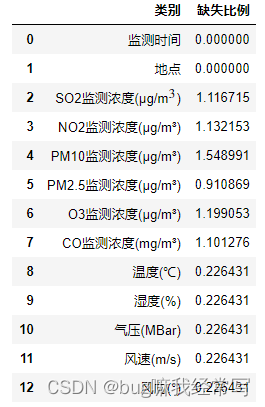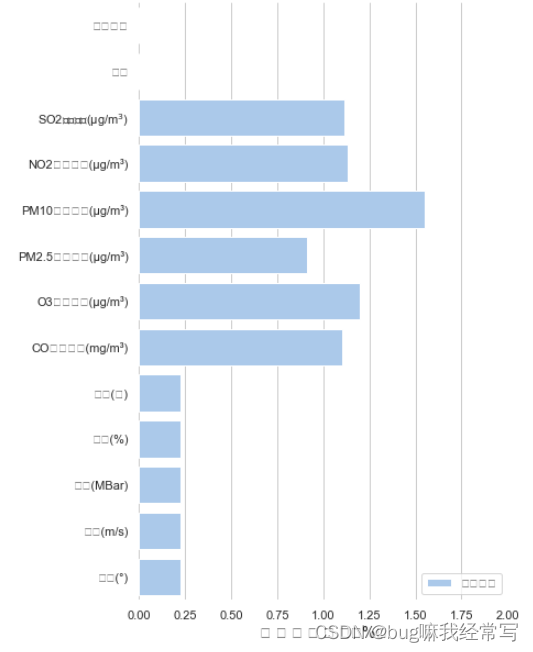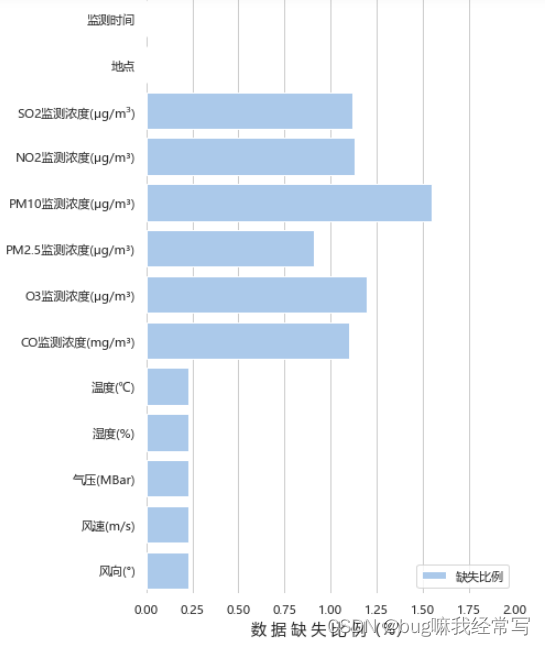# 问题描述

使用python seaborn绘制图表时，如果使用默认设置，那么会发现诸如汉字，上下角标的文字会出现乱码：

## 例如：下面用seaborn画一个柱状图

用到的数据格式如下：# 问题复现

问题代码：

import seaborn as sns
import matplotlib.pyplot as plt

sns.set_theme(style="whitegrid")

# 画布设置
f, ax = plt.subplots(figsize=(6, 10))

# 绘图
sns.set_color_codes("pastel")                          #设置色调
sns.barplot(x="缺失比例", y="类别", data=data,
label="缺失比例", color="b")
ax.legend(ncol=1, loc="lower right", frameon=True)    # 添加图例
ax.set(xlim=(0, 2), ylabel="")
plt.xlabel("数 据 缺 失 比 例（%）", fontsize=14)      # 调整横轴标题字体
sns.despine(left=True, bottom=True)                   # 移除坐标轴的线


运行效果：# 解决方法

sns.set_style(rc= {'font.sans-serif':"Microsoft Yahei"})，添加到原代码中。

import seaborn as sns
import matplotlib.pyplot as plt

sns.set_theme(style="whitegrid")
sns.set_style(rc={'font.sans-serif':"Microsoft Yahei"})
# 画布设置
f, ax = plt.subplots(figsize=(6, 10))

# 绘图
sns.set_color_codes("pastel")                          #设置色调
sns.barplot(x="缺失比例", y="类别", data=data,
label="缺失比例", color="b")
ax.legend(ncol=1, loc="lower right", frameon=True)    # 添加图例
ax.set(xlim=(0, 2), ylabel="")
plt.xlabel("数 据 缺 失 比 例（%）", fontsize=14)      # 调整横轴标题字体
sns.despine(left=True, bottom=True)                   # 移除坐标轴的线12-106031
10-301927
04-081985
07-122446
12-161654
05-303186
03-114739
03-04886
01-262320
11-21473
07-075398

### “相关推荐”对你有帮助么？

•非常没帮助
•没帮助
•一般
•有帮助
•非常有帮助被折叠的  条评论 为什么被折叠?到【灌水乐园】发言bug嘛我经常写

¥2 ¥4 ¥6 ¥10 ¥20余额支付 (余额：-- )扫码支付获取中扫码支付点击重新获取扫码支付1.余额是钱包充值的虚拟货币，按照1:1的比例进行支付金额的抵扣。
2.余额无法直接购买下载，可以购买VIP、C币套餐、付费专栏及课程。余额充值